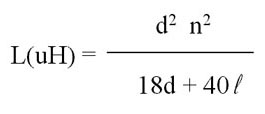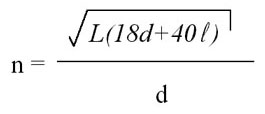Air Coil Calculation

This page will explain how to calculate coils and will explain how you can make your own coils.
I have made coils with different number of turns and measured the inductance.

Formula :Where:
L = inductance in micro henrys
D = coil diameter in inches (from wire center to wire center)
l = coil length in inches ( 1 inches = 25.4 mm)
n = number of turns

There are lots of Internet-pages explaining how to make coils, but there are few that will give you exact values. I have been experimenting with different diameters, length and wire-diameter and found one good combination.

To make the coils, I have used a two drills with a diameter of 7.2mm and 5.8mm. The diameter is chosen to give wide range of inductance and the coils will get good Q-values. The wire for the coils has a diameter of 0.6mm. The diameter of the wire is not so critical. The tables below shows the results.

 INDUCTANCE TABLE (diameter 7.2 mm, 0.6mm wire) Number of turns Inductance (nH) (Compact coil) Q-value 13-MHz (Compact coil) Inductance (nH) (Air-space coil) Q-value 13-MHz (Air-space coil) 3 77 407 66 440 4 122 325 102 560 5 177 340 - - 6 240 440 206 550 7 306 509 290 690 8 379 607 319 1300 9 470 1500 422 >1500 10 582 >1000 515 >1000 11 644 >1000 - >1000 12 656 >1000 545 >1000 13 745 >1000 612 >1000 14 789 >1000 658 >1000

 INDUCTANCE TABLE (diameter 5.8 mm, 0.6mm wire) Number of turns Inductance (nH) (Compact coil) Q-value 13-MHz (Compact coil) Inductance (nH) (Air-space coil) Q-value 13-MHz (Air-space coil) 4 92 540 79 - 5 131 370 120 530 6 175 340 155 500 7 220 300 184 640 8 272 370 234 560 9 315 470 267 770 10 363 650 313 1270

The coil can be compact with no space between the winding or you can pull it out, to make some space between the windings. My measurements shows that you will get the best Q-value if you pull out the winding so the air-space is 0.6mm between the turns in the winding. The inductance will drop a little, se the table above.

* Compact = There is no space between the windings in the coil!
* Air space= There is an air-space between the winding with the same length as the diameter of the wire you are using (0.6mm in this case).

73s

VU2FD